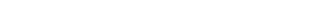## # 1.算法

• 时间：执行当前算法所消耗的时间，我们通常用「时间复杂度」来描述。
• 空间：执行当前算法需要占用多少内存空间，我们通常用「空间复杂度」来描述。

## # 2.时间复杂度

• 事后统计的方法：编写算法程序后再进行分析

• 事前估算的方法：通过分析算法的时间复杂度来判断算法的优劣

### # 2.1 时间频度

// 方式 1：使用 for 循环，一共执行了 2n+2 次
int total = 0;                 // 执行 1 次
for (int i = 1; i <= n; i++) { // 执行 n+1 次
total += i;                // 执行 n 次
}

// 方式 2：公式直接计算，一共执行了 2 次
int total = 0;            // 执行 1 次
total = (1 + n) * n / 2;  // 执行 1 次
}


### # 2.2 时间复杂度的定义

#### # 2.2.1 忽略项

$T(n)=3n+10$$T_1(n)=3n$ 随着 $n$ 增大，两条函数曲线无限接近，常数项 10 可忽略。

$T(n)=2n^2+3n+10$$T_1(n)=2n^2$ 随着 $n$ 增大，两条函数曲线无限接近，低次项 $3n+10$ 可忽略。

$T(n)=5n^2+7n$$T_1(n)=3n^2 + 2n$ 随着 $n$ 增大，两条函数曲线无限接近，$T(n)$ 系数 5 与 $T_1(n)$ 系数 3 可忽略。

#### # 2.2.2 计算时间复杂度的方法

1. 用常数 1 代替运行时间中的所有加法常数为：$3n^2+7n+1$

2. 修改后的运行次数函数中，只保留最高阶项为：$3n^2$

3. 去除最高阶项的系数为：$n^2$

#### # 2.2.3 常见的时间复杂度

• 常数阶 $O(1)$

• 对数阶 $O(\log_2n)$

• 线性阶 $O(n)$

• 线性对数阶 $O(n\log_2n)$

• 平方阶 $O(n^2)$

• 立方阶 $O(n^3)$

• k 次方阶 $O(n^k)$

• 指数阶 $O(2^n)$

int i = 1;
int j = 2;
++i;
j++;
int m = i + j;


for (i = 1; i <= n; ++i) {
j = i;
j++;
}


int i = 1;
while (i < n) {
i = i * 2;
}


for (i = 1; i <= n; ++i) {
i = 1;
while (i < n) {
i = i * 2;
}
}


for (i = 1; i <= n; i++) {
for (j = 1; j <= n; j++) {
a = i + j;
}
}


### # 2.3 平均时间复杂度和最坏时间复杂度

• 所有可能的输入实例均以等概率出现的情况下，该算法的运行时间，称为平均时间复杂度。

• 最坏情况下的时间复杂度称最坏时间复杂度。

## # 3.空间复杂度

• 算法本身占用的空间，输入、输出、指令、常数、变量等

• 算法要使用的辅助空间

• 对一个算法在运行过程中临时占用存储空间大小的量度。

• 空间复杂度是关于 $n$ 的函数，随着 $n$ 的增大，占用的存储越大。

## # 4.算法复杂度指南

### # 4.2 常用数据结构算法复杂度

Θ(n) Θ(n) Θ(1) Θ(1) O(n) O(n) O(1) O(1) O(n)
Θ(n) Θ(n) Θ(1) Θ(1) O(n) O(n) O(1) O(1) O(n)

B 树 Θ(log(n)) Θ(log(n)) Θ(log(n)) O(log(n)) O(log(n)) O(log(n)) O(log(n)) O(log(n)) O(n)

AVL Tree Θ(log(n)) Θ(log(n)) Θ(log(n)) Θ(log(n)) O(log(n)) O(log(n)) O(log(n)) O(log(n)) O(n)
KD Tree Θ(log(n)) Θ(log(n)) Θ(log(n)) Θ(log(n)) O(n) O(n) O(n) O(n) O(n)

### # 4.3 排序算法-算法复杂度

Timsort Ω(n) Θ(n log(n)) O(n log(n)) O(n)

Tree Sort Ω(n log(n)) Θ(n log(n)) O(n^2) O(n)

Cubesort Ω(n) Θ(n log(n)) O(n log(n)) O(n)

## # 参考